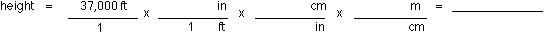+ Text Only Site
+ Non-Flash Version
+ Contact GlennAircraft Motion (Level 1) Answers   What is the smallest angle in degrees between the lift and drag forces? (a) 0o (b) 90o (c) 180o (d) 270o (e) 360o  What is the smallest angle in degrees between the weight and lift forces? (a) 0o (b) 90o (c) 180o (d) 270o (e) 360o What is the smallest angle in degrees between the thrust and drag forces? (a) 0o (b) 90o (c) 180o (d) 270o (e) 360o What is the smallest angle in degrees between the weight and thrust forces? (a) 0o (b) 90o (c) 180o (d) 270o (e) 360o When an airplane (or any object) is moving at a constant speed, the forces acting on the airplane must be (a) unbalanced, which means that the total force is not zero. (b) balanced, which means that the total force is zero.  A fighter jet is flying at 37,000 feet with a constant speed of 1,310 km/h. The jet has a weight of 110,000 N and its engines provide a thrust of 106,752 N (using afterburners).  What is the jet's height (altitude) in meters?Step 1: Recall that 12 inches = 1 foot, 2.54 cm = 1 inch, and 100 cm = 1 meter. Step 2: Write these unit factors in their appropriate places in the factor multiplication. (a) 1.13 x 108 m (b) 61,248 m (c) 8,679 m (d) 356 m (e) 11,277.6 m What is the jet's mass? [Hint: Use F = mag = W, where ag = 9.8 m/s2.] Step 1: force = weight = 110,000 N; a = 9.8 m/s2 (acceleration due to gravity) Step 2: mass = force / acceleration (a) 520,120 kg (b) 11,224.49 kg (c) 3,527 kg (d) 614,853 kg (e) 215 kg How much lift force is being applied to the jet? Realize that if the airplane's speed is constant, the forces acting on the airplane must be balanced. Force of lift = Force of weight (a) 512,128 N (b) 5,634 N (c) 110,000 N (d) 9,935 N (e) 106,752 N   If the jet engines are creating a thrust of 106,752 N, what is the drag force applied to the jet? Realize that if the airplane's speed is constant, the forces acting on the airplane must be balanced. Force of drag = Force of thrust (a) 512,128 N (b) 5,634 N (c) 110,000 N (d) 9,935 N (e) 106,752 N   Suppose the jet engines are providing a thrust of 100,102 N while the drag force is only 90,167 N.   What is the total unbalanced (or net) force on the jet? Step 1: Fthrust = 100,102 N; Fdrag = 90,167 N Step 2: Ftotal = Fthrust - Fdrag ( Fnet = Ftotal) (a) 512,128 N (b) 5,634 N (c) 110,000 N (d) 9,935 N (e) 106,752 N   What will be the jet's acceleration? Step 1: F = 9,935 N; m = 11,224.49 kg Step 2: acceleration = force / mass (a) 0.89 m/s2 (b) 4.35 m/s2 (c) 0.36 m/s2 (d) 4,253 m/s2 (e) 65.3 m/s2   If the jet is cruising at 223.47 m/s and accelerates at 0.25 m/s2 for 1,530 seconds, what is the jet's final speed? Step 1: vi = initial velocity = 223.47 m/s; a = 0.25 m/s2; t = 1,530 sec (vf = final velocity) Step 2: a = ( vf - vi ) / t a) 401.28 m/s (b) 605.97 m/s (c) 731.67 m/s (d) 634,521.6 m/s (e) 3,452 m/s   Using the data from the previous problem, calculate how far the jet traveled while it accelerated during the 1,530 seconds. Step 1: vi = 223.47 m/s; a = 0.25 m/s2; t = 1,530 sec; di = initial distance = 0 m (df = final distance) Step 2: df = ( 1 / 2 ) at2 + vit + di (a) 401.28 m (b) 605.97 m (c) 731.67 m (d) 634,521.6 m (e) 3,452 m   If the jet cruised at a speed of 1,310 km/h for 2.53 hours, how far did it travel? Step 1: v = 1310 km/hr.; t = 2.53 hr. Step 2: d = vt (a) 517.79 km (b) 4,639.00 km (c) 218.42 km (d) 6,547.23 km (e) 3,314.30 km

Related Pages:
Standards
Activity
Worksheet
Lesson Index
Aerodynamics Index+ Inspector General Hotline + Equal Employment Opportunity Data Posted Pursuant to the No Fear Act + Budgets, Strategic Plans and Accountability Reports + Freedom of Information Act + The President's Management Agenda + NASA Privacy Statement, Disclaimer, and Accessibility CertificationEditor: Tom Benson NASA Official: Tom Benson Last Updated: Thu, May 13 02:38:26 PM EDT 2021 + Contact Glenn